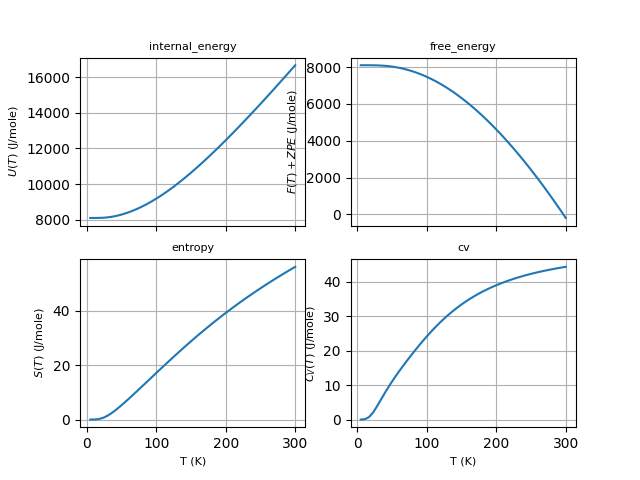# Thermodinamic properties¶

This example shows how to compute and plot thermodinamic properties within the harmonic approximation using the phonon DOS produced by anaddb.

from abipy.abilab import abiopen
import abipy.data as abidata

# Read the Phonon DOS from the netcd file produced by anaddb (prtdos 2)
ncfile = abiopen(abidata.ref_file("trf2_5.out_PHDOS.nc"))
phdos = ncfile.phdos

# Print crystalline structure and zero-point energy.
print(ncfile.structure)
zpe = phdos.zero_point_energy
print("Zero point energy:", zpe, zpe.to("J"), zpe.to("Ha"))


Out:

Full Formula (Al1 As1)
Reduced Formula: AlAs
abc   :   3.970101   3.970101   3.970101
angles:  60.000000  60.000000  60.000000
Sites (2)
#  SP       a     b     c
---  ----  ----  ----  ----
0  Al    0     0     0
1  As    0.25  0.25  0.25

Abinit Spacegroup: spgid: 0, num_spatial_symmetries: 24, has_timerev: True, symmorphic: False
Zero point energy: 0.08400926480898568 eV 1.3459768111647533e-20 J 0.0030872835382053307 Ha


Compute free energy from 2 to 300 K (20 points) By default, energies are is eV and thermodynamic quantities are given on a per-unit-cell basis.

f = phdos.get_free_energy(tstart=2, tstop=300, num=20)
#f.plot()


Plot U, F, S, Cv as a function of T. Use J/mol units, results are divided by formula_units.

phdos.plot_harmonic_thermo(units="Jmol", formula_units=1)Out:

<Figure size 640x480 with 4 Axes>


Plotly version:

phdos.plotly_harmonic_thermo(units="Jmol", formula_units=1)


Remember to close the file:

ncfile.close()


Total running time of the script: ( 0 minutes 0.353 seconds)

Gallery generated by Sphinx-Gallery